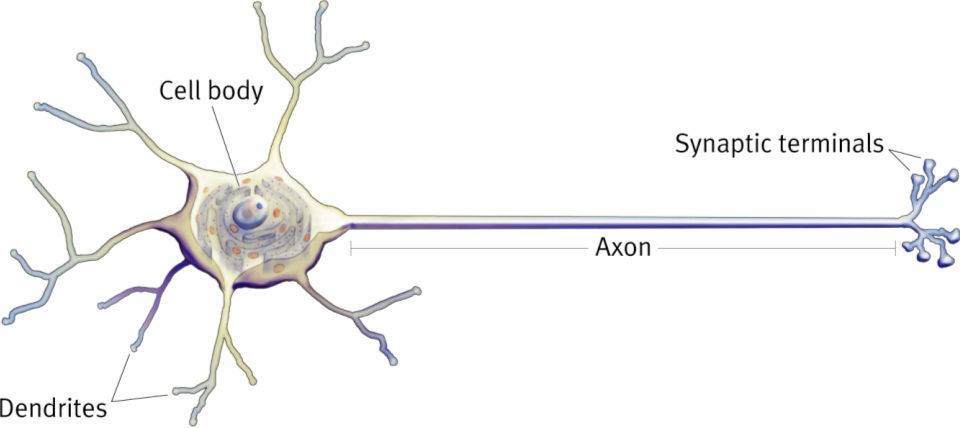top of page

# The Perceptron Algorithm

The Perceptron Algorithm is one of the oldest Machine Learning algorithm developed in 1950's.

The motivation behind this algorithm comes from the study of a biological neuron in order to replicate the intelligence brain to develop Artificial Intelligence.This is how a neuron works -

A neuron takes input signals from its surroundings using Dendrites

and send to the cell body. Once the received signal crosses the threshold point, the neuron gets fired/activated and passes the signal via Axon to the Synaptic terminals..

A basic Perceptron works on the same principle. It is used in binary classification problems,

Fig. 1 NeuronJust as we can see the figure of a perceptron, we pass the inputs via the some weights. And then we sum the product of the weights and the inputs. Weights are something that we learn overtime from the data that is given to us. That part is the "Learning" in Machine Learning, This whole summation is passed to an activation function which generates probability of a class in binary classification.

How we learn weights -

A Perceptron seeks to minimize the loss function which is defined as -

L = - Σ (yi * (xi^T * w))

which is summation of y and the product of x and w over all the data points where y is not same as that of sign of x*w.

By minimizing L, we try to predict the correct label.

After calculating the loss function using the above formula, we update the weights using the below equation.

W(new) = W(old) - ( η * ΔL )

Here, ΔL cannot be solved analytically, but it gives us a sense of direction in which L is increasing in w. η is a constant and also called learning rate. It sets the speed with which we learn.

The Loss calculated using the W(new) is less than the loss calculated using W(old). This procedure is one step of optimizing the objective function. After repeating it for certain number of times, we get the value of W that fits well to the data and produces a low loss. This is procedure of updating the value of weights using the delta of the loss function is called Gradient Descent.

And after multiple iterations of Gradient Descent, we have the optimized value of W which is then used to predict the class of the incoming data.

One thing to notice about Perceptron is that if the data is not linearly separable, then its not possible to converge the value of W using Gradient Descent, which leads to the bad fit and wrong predictions.

Kush.

bottom of page